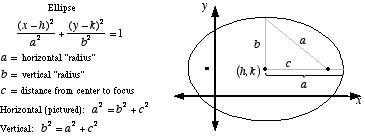index: click on a letter A B C D E F G H I J K L M N O P Q R S T U V W X Y Z A to Z index index: subject areas numbers & symbols sets, logic, proofs geometry algebra trigonometry advanced algebra & pre-calculus calculus advanced topics probability & statistics real world applications multimedia entrieswww.mathwords.com about mathwords website feedback

 Foci of an Ellipse Two fixed points on the interior of an ellipse used in the formal definition of the curve. An ellipse is defined as follows: For two given points, the foci, an ellipse is the locus of points such that the sum of the distance to each focus is constant. Note: If the interior of an ellipse is a mirror, all rays of light emitting from one focus reflect off the inside and pass through the other focus.See also